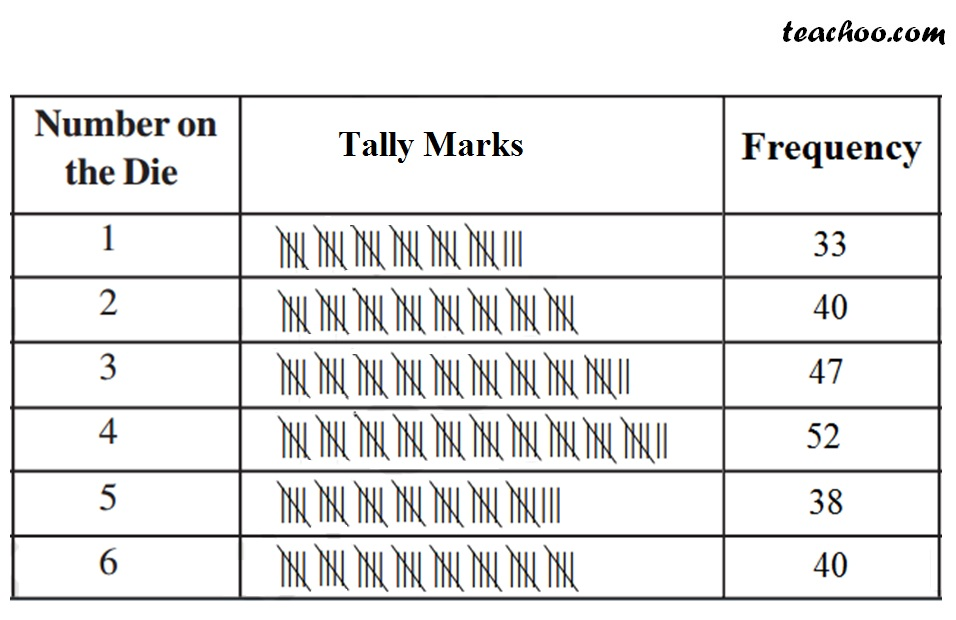Probability

Chapter 3 Class 7 Data Handling
Concept wise

Suppose we have the following statements

1. Sun rises from the west
2. Ganga is the river in India
3. India will win the test match

Statement 1 is always false.

as Sun rises from the east

Statement 2 is always true.

as Ganga is a river in India

Statement 3 is not always true or false.

India can win the match or not. Both are possible.

In statement 3, we can try to find the chance where India can win

Let’s look at Chance & Probability

#### Chance

Suppose we toss a coin 25 times.

Our result can be something like

Hence,

Chance of Tail Coming = (Total number of times Tail has come)/(Total number of outcomes)

= 16/25

Chance of Head Coming = (Total number of times Head has come)/(Total number of outcomes)

= 9/25

Chance is defined by the result of outcomes.

But, what if we toss the coin

1000 times

or  10000 times

or   Infinite times?

Assuming the number of outcomes is infinite,

then chance becomes Probability

Similarly,

we can do for a die

## A die is thrown 250 times, whose outcomes are represented below. What will be the probability of getting 5?Hence,

Chance of getting 5 = 38/250

#### Probability

Suppose we toss a coin,

there are only 2 possible outcomes − Heads or Tails

= (Number of outcome where head can come)/(Total possible outcomes numbers)

= 1/2

Similarly,

Probability of getting Tail = 1/2

Similarly for Die,

Probability outcomes = 1, 2, 3, 4, 5, 6

Probability of getting 5

= (Number of outcome where 5 can come)/(Total possible outcomes numbers)

= 1/6

Probability of getting 7 = 0/6

= 0

Probability of getting number less than or equal to 6 = 6/6

= 1

Learn in your speed, with individual attention - Teachoo Maths 1-on-1 Class